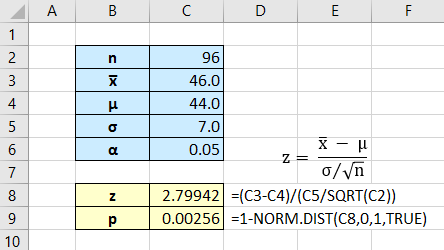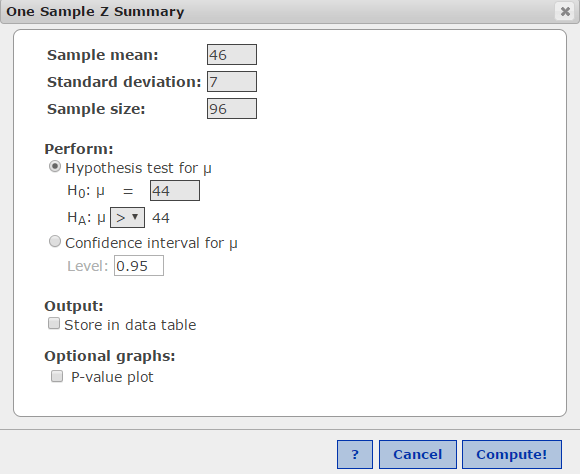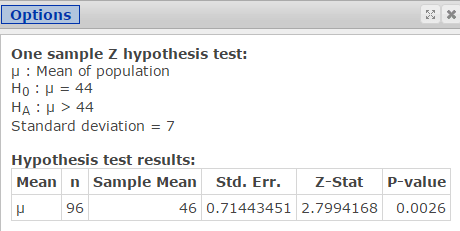# Normal Distribution: Example Problem 1

One way to improve your ability to solve normal distribution problems is to work on recognizing what word problems are requesting you to do.

Consider the following problem statement:

In an investigation of the personality characteristics of drug dealers of a certain region, convicted drug dealers were scored on a scale that provides a quantitative measure of person’s level of need for approval and sensitivity to social situations. (Higher scores indicate a greater need for approval.) Based on the study results, it can be assumed that the scale scores for the population of convicted drug dealers of the region has a mean of 44 and a standard deviation of 7. Suppose that in a sample of 96 people from the region, the mean scale score is x̅ = 46. Is this sample likely to have been selected from the population of convicted drug dealers of the region? Explain. Consider an event with a probability less than 0.05 unlikely. (McClave, Benson, & Sincich, 2014)

Solution:

First, state the question: How unusual would it be to get a sample mean of 46 if the population mean is 44 and the population standard deviation is 7?

In math form, find P(x̅ ≥ 46) for µ = 44, σ = 7.  Note: use the ≥ math operator because we need to find the area under the normal curve to the right of the sample mean. Because the normal distribution is continuous, the probability of getting exactly 46 is meaningless.

Second, identify the data provided:3rd, draw a sketch. You can see that x̅ = 46 falls far to the right of the mean, µ = 44.Excel solution:Because p < α =0.05, it would be unusual to get a randomly collected sample mean of 46 or greater if the population mean is 44 and the population standard deviation is 7.

StatCrunch solution: Use the command sequence Stats > Z Stats > One Sample > With Summary. Enter the sample mean, the standard deviation, and n. (Note: enter the population standard deviation, σ; StatCrunch calculates the sample standard error.)

Select the radio button next to Hypothesis test for µ, enter the null value of 44, and select > for the alternative Ha. Click Compute!We get the same results as we did with Excel.This site uses Akismet to reduce spam. Learn how your comment data is processed.